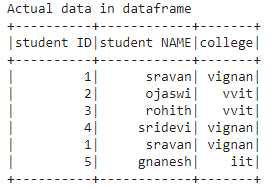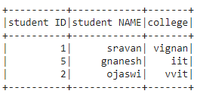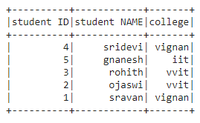# Removing duplicate rows based on specific column in PySpark DataFrame

• Last Updated : 06 Jun, 2021

In this article, we are going to drop the duplicate rows based on a specific column from dataframe using pyspark in Python. Duplicate data means the same data based on some condition (column values). For this, we are using dropDuplicates() method:

Syntax: dataframe.dropDuplicates([‘column 1′,’column 2′,’column n’]).show()

where,

• dataframe is the input dataframe and column name is the specific column
• show() method is used to display the dataframe

Let’s create the dataframe.

## Python3

 `# importing module``import` `pyspark`` ` `# importing sparksession from pyspark.sql``# module``from` `pyspark.sql ``import` `SparkSession`` ` `# creating sparksession and giving an app name``spark ``=` `SparkSession.builder.appName(``'sparkdf'``).getOrCreate()`` ` `# list  of students  data``data ``=` `[[``"1"``, ``"sravan"``, ``"vignan"``], [``"2"``, ``"ojaswi"``, ``"vvit"``],``        ``[``"3"``, ``"rohith"``, ``"vvit"``], [``"4"``, ``"sridevi"``, ``"vignan"``], ``        ``[``"1"``, ``"sravan"``, ``"vignan"``], [``"5"``, ``"gnanesh"``, ``"iit"``]]`` ` `# specify column names``columns ``=` `[``'student ID'``, ``'student NAME'``, ``'college'``]`` ` `# creating a dataframe from the lists of data``dataframe ``=` `spark.createDataFrame(data, columns)`` ` `print``(``'Actual data in dataframe'``)``dataframe.show()`

Output:Dropping based on one column

## Python3

 `# remove duplicate rows based on college ``# column``dataframe.dropDuplicates([``'college'``]).show()`

Output:Dropping based on multiple columns

## Python3

 `# remove duplicate rows based on college ``# and ID column``dataframe.dropDuplicates([``'college'``, ``'student ID'``]).show()`

Output:My Personal Notes arrow_drop_up